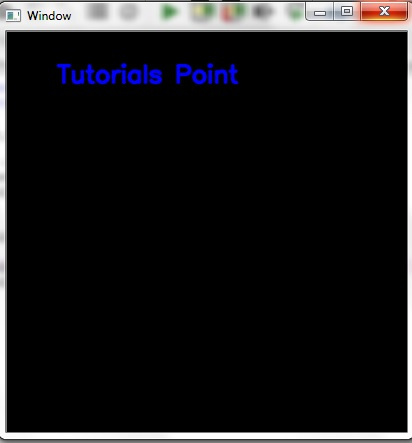# Draw geometric shapes on images using Python OpenCv module

The basic operations of OpenCV is to draw over images. The ability to add different geometric shapes just like lines, circles and rectangle etc.

Often working with image analysis, we want to highlight a portion of the image, for example by adding a rectangle that defines that portion. Also as example an arrow to indicate something.

cv2.line() − This function is used to draw line on an image.

cv2.rectangle() − This function is used to draw rectangle on an image.

cv2.circle() − This function is used to draw circle on an image.

cv2.putText() − This function is used to write text on image.

cv2.ellipse() − This function is used to draw ellipse on image.

## Example code

import numpy as np
import cv2
my_img = np.zeros((350, 350, 3), dtype = "uint8")
cv2.imshow('Window', my_img)
cv2.waitKey(0)
cv2.destroyAllWindows()


## Output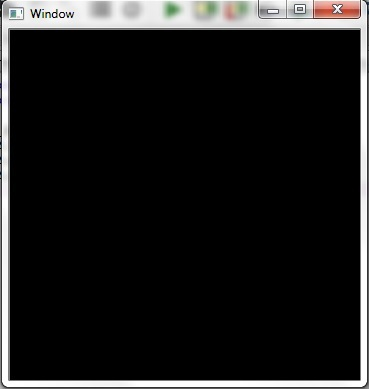## To draw a Line

For drawing a line cv2.line() function is used. This function takes five arguments

• Image object on which to draw
• Starting point coordinates (x, y)
• End point coordinates (x, y)
• Stroke color in BGR (not RGB, to be noted)
• Stroke thickness (in pixels)

## Example code

import numpy as np
import cv2
my_img = np.zeros((350, 350, 3), dtype = "uint8")
# creating for line
cv2.line(my_img, (202, 220), (100, 160), (0, 20, 200), 10)
cv2.imshow('Window', my_img)
cv2.waitKey(0)
cv2.destroyAllWindows()


## Output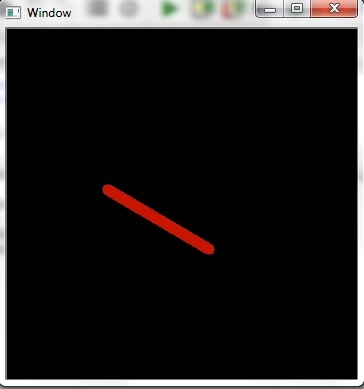## To draw a Rectangle

For drawing a rectangle cv2.rectangle() function is used. This function accepts five input parameters.

• Image object on which to draw
• Coordinates of the vertex at the top left (x, y)
• Coordinates of the lower right vertex (x, y)
• Stroke color in BGR (not RGB, to be noted)
• Stroke thickness (in pixels)

## Example code

import numpy as np
import cv2
my_img = np.zeros((400, 400, 3), dtype = "uint8")
# creating a rectangle
cv2.rectangle(my_img, (30, 30), (300, 200), (0, 20, 200), 10)
cv2.imshow('Window', my_img)
# allows us to see image
# until closed forcefully
cv2.waitKey(0)
cv2.destroyAllWindows()


## Output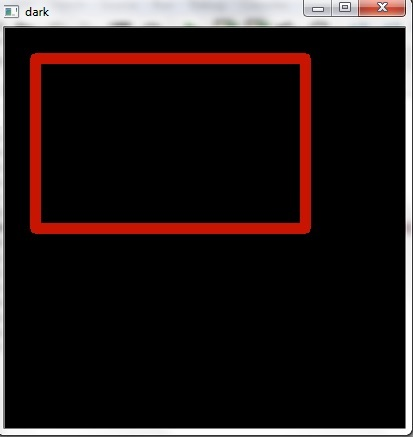## To draw a circle

For drawing a circle, cv2.circle() function is used. This function accepts five input parameters.

• Image object on which to draw
• Center coordinates (x, y)
• Stroke color in BGR (not RGB, to be noted)
• Stroke thickness (in pixels)

## Example code

import numpy as np
import cv2
my_img = np.zeros((400, 400, 3), dtype = "uint8")
# creating circle
cv2.circle(my_img, (200, 200), 80, (0, 20, 200), 10)
cv2.imshow('Window', my_img)
cv2.waitKey(0)
cv2.destroyAllWindows()


## Output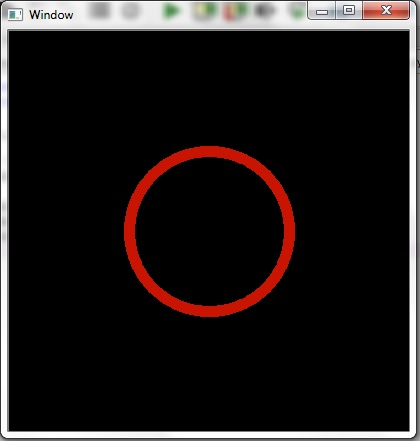## To draw a ellipse

For drawing an ellipse, cv2.ellipse() function is used. This function accepts eight input parameters.

• Image object on which image to be drawn
• Center coordinates (x, y)
• Length of the minor and major axes (h, w)
• Rotation angle of the ellipse (calculated counterclockwise)
• Starting angle (calculated clockwise)
• Final angle (calculated clockwise)
• Stroke color in BGR (not RGB to be noted)
• Stroke thickness

## Example code

import numpy as np
import cv2
my_img = np.zeros((400, 400, 3), dtype = "uint8")
# creating for rectangle
cv2.ellipse(my_img,(256,256),(100,50),0,0,180,255,-1)
cv2.imshow('Window', my_img)
# allows us to see image
# until closed forcefully
cv2.waitKey(0)
cv2.destroyAllWindows()


## Output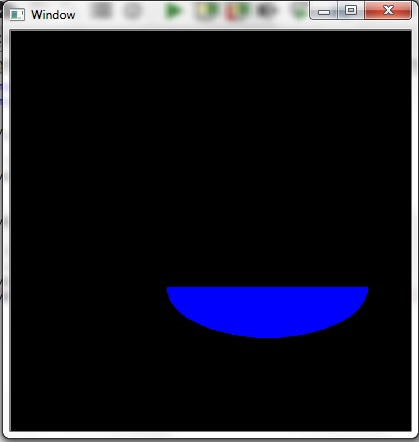## To draw a polygon

For drawing a polygon, cv2.polylines() function is used. This function needs five number of arguments.

• The image object on which to draw
• The array of coordinates
• True, if it is a closed line
• Stroke color
• Stroke thickness

## Example code

import numpy as np
import cv2
my_img = np.zeros((400, 400, 3), dtype = "uint8")
pts = np.array([[10,5],[20,30],[70,20],[50,10]], np.int32)
pts = pts.reshape((-1,1,2))
cv2.polylines(my_img,[pts],True,(0,255,255))
cv2.imshow('Window', my_img)
cv2.waitKey(0)
cv2.destroyAllWindows()


Output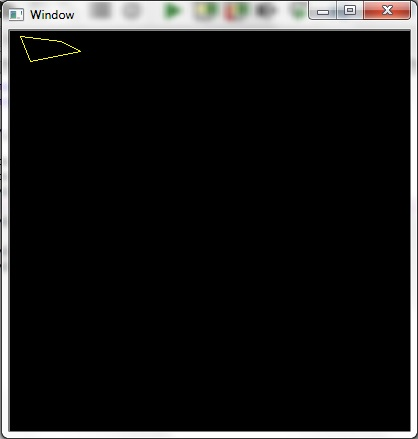## To draw the text

To write text with OpenCV there is cv2.putText() function that accepts a number of arguments.

• The image on which to draw
• The text to be written
• Coordinates of the text start point
• Font to be used
• Font size
• Text color
• Text thickness
• The type of the line used

## Example code

import numpy as np
import cv2
my_img = np.zeros((400, 400, 3), dtype = "uint8")
# Writing text
font = cv2.FONT_HERSHEY_SIMPLEX
cv2.putText(my_img, 'Tutorials Point', (50, 50),font, 0.8, (255, 0, 0), 2, cv2.LINE_AA)
cv2.imshow('Window', my_img)
cv2.waitKey(0)
cv2.destroyAllWindows()


## Output